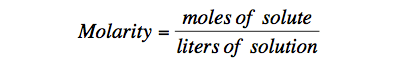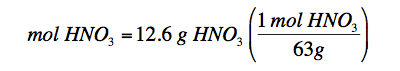# Molarity 126

## How to Solve Molarity Problems

Molarity is a quantitative description of solution concentration. It is abbreviated M.Using the formula above and your basic knowledge of algebra, solve the following problems:

Example 1

To make a 4.00 M solution, how many moles of solute will be needed if 12.0 liters of solution are required?

Step 1:

Identify each piece of information given in the problem and identify what the problem is asking for.

M = 4.00 M

mol = ?

L = 12.0 L

Step 2:

Rearrange the molarity equation to solve it for the variable that the question is asking for.

moles of solute = Molarity x liters of solution

(Multiply both sides of the equation by liters of solution. On the right hand side of the equation, they cancel out, on the other side, you are left with Molarity x liters of solution.)

Step 3:

Plug in your data from step 1 into the rearranged equation from step 2.

moles of solute = 4.00 M x 12.0 L

moles of solute = 48.0 mol

Remember that M stands for mol / L. If we substitute this into the equation, we can see that L will cancel, leaving units of mol.

You try this one:

Roll your cursor over the words Hint, Step 1, Step 2, and then Step 3 to see the solutions and explanations.

How many moles of sucrose are dissolved in 250 mL of solution if the solution concentration is 0.150 M?

Hint

Notice that your volume is in mL. It must be converted to L to be used in this equation.

Step 1

M = 0.150 M
mol = ?
L = 250 mL (1L/1000 mL) = 0.25 L

Step 2

moles of solute = Molarity x liters of solution

Step 3

M = 0.150 M
mol = ?
L = 250 mL (1 L / 1000 mL) = 0.25 L
moles of solute = Molarity x liters of solution
moles of solute = 0.150 M x 0.25 L
moles of solute = 0.038 mol

Example 2

What is the molarity of a solution of HNO3 that contains 12.6 grams HNO3 in 1.0 L of solution?

Step 1:

Identify each piece of information given in the problem and identify what the problem is asking for.

M = ?

mol = ?

L = 1.0 L

In this equation moles are not given, but grams are. You must use your knowledge of molar mass to convert grams of HNO3 to moles.mol HNO3 = 0.200 mol

Step 2:

Rearrange the molarity equation to solve it for the variable that the question is asking for.

Molarity = moles of solute/liters of solution

Step 3:

Plug in your data from step 1 into the rearranged equation from step 2.

M = 0.200 mol HNO3 / 1.0 L

M = 0.200 M

You try this one:

Click on the words Step 1, Step 2, and then Step 3 to see the solutions and explanations.

How many grams of potassium nitrate are required to prepare 0.250 L of a 0.700 M solution?

Step 1

M = 0.700 M
mol = ?
liters = 0.250 L
Notice the question is asking for grams of potassium nitrate. We must first solve for moles, then use what we know about molar mass to solve for grams. Additionally, we msut know the formula for potassium nitrate. Potassium forms a +1 ion, because it is in group 1 with 1 valence electron. Nitrate is one of those polyatomic ions that you were supposed to learn in a previous lesson. The formula is NO3-1. The formula for potassium nitrate is KNO3.

Step 2

mol = M x liters

Step 3

mol KNO3 = 0.700 M x 0.250 L
mol KNO3 = 0.175 mol
g KNO3 = 0.175 mol KNO3 x (101.1 g KNO3 / 1 mol KNO3 )
g KNO3 = 17.7 g KNO3

Example 3

125 cm3 of solution contains 3.5 moles of solute. What is the molarity of the solution?

Step 1:

Identify each piece of information given in the problem and identify what the problem is asking for.

M = ?

mol = 3.5 mol

L = ?

In this equation, the volume is given in cm3. We know from a previous module that 1 cm3 = 1 mL. You must use your knowledge of metric prefixes to complete this conversion.Step 2:

Rearrange the molarity equation to solve it for the variable that the question is asking for.

Molarity = moles of solute/ liters of solution

Step 3:

Plug in your data from step 1 into the rearranged equation from step 2.

M = 3.5 mol / 0.125 L

M = 28 M

source